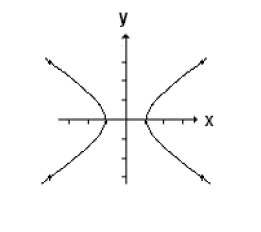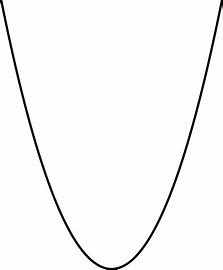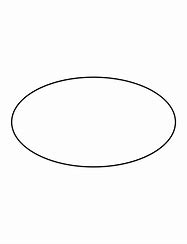Courses
Courses for Kids
Free study material
Free LIVE classes
More

# Difference Between Hyperbola Parabola and EllipseLIVE
Join Vedantu’s FREE Mastercalss

## What is Hyperbola and Ellipse and Parabola: Introduction

To explain hyperbola and ellipse and parabola: A hyperbola is defined by the difference in distances between any point on the curve and two fixed points called the foci. It has two distinct branches that never intersect. A parabola is formed by all points equidistant from a single focus and a directrix. It has a symmetrical shape and extends infinitely. An ellipse is a closed curve formed by all points where the sum of distances to two fixed points, the foci, is constant. It can vary from a circle to a stretched or compressed oval. These conic sections have unique properties and find applications in various fields, including optics, astronomy, and engineering. Read further for more characteristics of hyperbola parabola and ellipse.

### Defining Hyperbola

A hyperbola is a conic section with two distinct branches that never intersect. It is defined by two foci and the difference of distances between any point on the curve and the foci is constant. Hyperbolas have asymptotes, which are straight lines that the branches approach but never touch. They are characterized by their eccentricity, asymmetry, and the presence of a transverse and conjugate axis. Hyperbolas find applications in mathematics, physics, and engineering. Some characteristics of the hyperbola are:

• Two branches: A hyperbola consists of two separate branches that are symmetric with respect to the center. These branches extend infinitely and never intersect.

• Foci: A hyperbola is defined by two fixed points called the foci. The difference in distances between any point on the hyperbola and the two foci is constant.

• Asymptotes: Hyperbolas have asymptotes, which are straight lines that the branches of the hyperbola approach but never touch. These asymptotes intersect at the center of the hyperbola and form a rectangular shape.

• Eccentricity: The eccentricity of a hyperbola determines its shape. It is the ratio of the distance between the center and one of the foci to the distance between the center and a point on the hyperbola. The eccentricity of a hyperbola is always greater than 1.

• Asymmetry: Unlike ellipses, hyperbolas are not symmetric about their axes. Each branch of the hyperbola has its own distinct shape and orientation.

### Defining Parabola

A parabola is a conic section that has a symmetrical U-shaped curve. It is formed by all points that are equidistant from a fixed point called the focus and a straight line called the directrix. The vertex of the parabola is the point where the curve is closest to the focus. Parabolas have applications in various fields, including physics, engineering, and optics. They exhibit unique properties, such as reflecting incoming parallel rays to a single focal point. Some characteristics of the parabola are:

• Symmetry: A parabola is symmetric about its axis of symmetry, which is a vertical line passing through its vertex.

• Focus and directrix: A parabola is defined by a focus, which is a fixed point, and a directrix, which is a fixed line. The parabola is formed by all points that are equidistant from the focus and the directrix.

• Vertex: The vertex of a parabola is the point where the curve reaches its minimum or maximum point. It lies on the axis of symmetry.

• Axis of symmetry: The axis of symmetry of a parabola is a vertical line passing through the vertex. It divides the parabola into two symmetrical halves.

• Opening: The direction in which a parabola opens depends on the orientation of its curve. It can open upward or downward.

### Defining Ellipse

An ellipse is a closed curve in mathematics that is formed by all points where the sum of distances to two fixed points, called the foci, is constant. It has a distinct oval shape and can range from a perfect circle to a stretched or compressed form. The center of the ellipse is the midpoint between the foci. Ellipses find applications in geometry, astronomy, engineering, and other fields where their unique properties and symmetry play a significant role. Some characteristics of the ellipse are:

• Foci: An ellipse is defined by two fixed points called the foci. The sum of distances from any point on the ellipse to the two foci is constant.

• Major and minor axis: An ellipse has a major axis, which is the longest diameter that passes through the center, and a minor axis, which is the shortest diameter perpendicular to the major axis.

• Center: The center of an ellipse is the midpoint of the major axis. It is equidistant from both foci.

• Symmetry: Ellipses possess symmetry about both the major and minor axes. The curve is identical on both sides of these axes.

• Asymptotes: In some cases, an ellipse can have asymptotes, which are lines that the curve approaches but never intersects.

### Parabola Hyperbola And Ellipse Difference

 S.No Category Hyperbola Parabola Ellipse 1. ShapeImage - an image of hyperbolaImage - an image of parabolaImage - an image of ellipse 2. Foci Two foci One focus Two foci 3. Symmetry No axis of symmetry Symmetric about the axis Symmetric about both axes 4. Opening Branches open in opposite directions Opens either upward or downward No specific opening 5. Eccentricity Greater than 1 Equal to 1 Less than 1 6. Asymptotes Has asymptotes No asymptotes No asymptotes (except in certain degenerate cases)

This table provides a general overview of the difference and characteristics of hyperbola and ellipse also parabola. Now, after reading this article you can easily distinguish between them.

### Summary

A hyperbola is defined by the difference in distances between any point on the curve and two fixed points called the foci. A parabola is a U-shaped curve with a single focus and a directrix. It is formed by all points equidistant from the focus and the directrix. An ellipse is a closed curve formed by all points where the sum of distances to two fixed points, the foci, is constant.

Last updated date: 26th Sep 2023
Total views: 3k
Views today: 0.03k

## FAQs on Difference Between Hyperbola Parabola and Ellipse

1. How many branches does a hyperbola have?

A hyperbola has two distinct branches. Each branch of the hyperbola extends infinitely and never intersects. The branches are symmetrical with respect to the center of the hyperbola. The center is the point equidistant from both branches. The two branches of the hyperbola open in opposite directions, creating a symmetrical curve. These branches diverge further away from the center as they extend, forming the characteristic shape of a hyperbola.

2. What are the foci of a parabola?

A parabola has one focus. The focus is a fixed point located on the axis of symmetry, equidistant from the directrix. It is the point where all incoming parallel rays reflect off the curve and converge. The focus plays a significant role in defining the shape and properties of the parabola. The distance between the focus and the vertex is known as the focal length, and it determines the overall size and curvature of the parabola. The focus is a key element in understanding the reflective property of parabolas and their various applications.

3. How is an ellipse different from a circle?

An ellipse differs from a circle primarily in terms of its shape. While a circle is perfectly symmetrical and has all points equidistant from its center, an ellipse is elongated and has two distinct radii: the major axis (longest diameter) and the minor axis (shortest diameter). The major and minor axes of an ellipse are perpendicular to each other. Consequently, the distances from the center to any point on the ellipse vary, unlike in a circle where they are always the same. This variation in distance gives an ellipse its characteristic oval shape.

4. What is the relationship between the foci and the shape of an ellipse?

The foci of an ellipse play a crucial role in defining its shape. The sum of the distances from any point on the ellipse to the two foci is constant. This constant sum determines the overall elongation or compression of the ellipse. When the foci are close together relative to the length of the major axis, the ellipse appears more circular. Conversely, when the foci are further apart, the ellipse becomes more elongated.

5. What is the reflection property of a parabola?

The reflection property of a parabola states that any incoming ray parallel to the axis of symmetry of a parabola will reflect off the curve and pass through the focus. This property is a result of the parabolic shape and the positioning of the focus. It means that all the rays parallel to the axis of symmetry will converge at the focus after reflection. This property is utilized in applications such as reflectors, antennas, and focusing devices, where parallel rays are redirected to a single focal point.